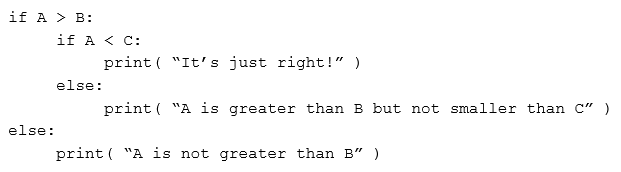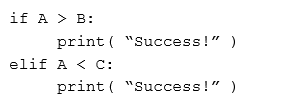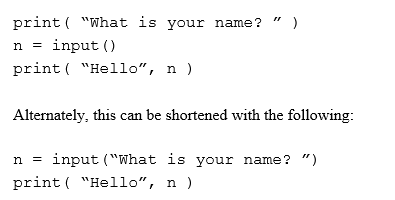# Python Learning Week 1

## Introduction to​​ Python

Background

Installing Python and JetBrains Pycharm:

https://www.jetbrains.com/pycharm/

Code Example 1 - Hello, World!

>>> print "Hello, World!“​​

Math in Python

>>> 1 + 1

2​​

>>> 6-5

1​​

>>> 2*5

10​​

>>> 5**2

25​​

>>> 21/3

7

>>> 23%3

2​​

Python Operators​​

 command​​ name​​ example​​ output​​ +​​ Addition​​ 4+5​​ 9​​ -​​ Subtraction​​ 8-5​​ 3​​ *​​ Multiplication​​ 4*5​​ 20​​ /​​ Division​​ 19/3​​ 6​​ %​​ Remainder​​ 19%3​​ 5​​ **​​ Exponent​​ 2**4​​ 16​​

Order of Operations:

• parentheses ()​​

• exponents **​​

• multiplication *, division \, and remainder %​​

• addition + and subtraction -​​

>>> #I am a comment. I can say whatever I want!​​

Variables:

print "This program is a demo of variables"

v = 1

print "The value of v is now", v

v = v + 1

print "v now equals itself plus one, making it worth", v

print "to make v five​​ times bigger, you would have to type v = v * 5"

v = v * 5

print "there you go, now v equals", v, "and not", v / 5​​

Strings:

word1 = "Good"​​

word2 = "Morning"​​

word3 = "to you too!"​​

print word1, word2​​

sentence = word1 + " " + word2 + " " +word3​​

print sentence​​

Basic Mathematics & Conditional Statements in Python

Boolean operators:

 Expression​​ Function​​ <​​ less than​​ <=​​ less that or equal to​​ >​​ greater than​​ >=​​ greater than or equal to​​ !=​​ not equal to​​ <>​​ not equal to (alternate)​​ ==​​ equal​​ to​​

Conditional Statements:

‘if' - Statement​​

y = 1

if y == 1:

​​ ​​ ​​​​ print "y still equals 1, I was just checking"

‘if - else' - Statement​​

a = 1

if a > 5:

​​ ​​ ​​​​ print "This shouldn't happen."

else:

​​ ​​ ​​​​ print "This should happen."

‘elif' - Statement​​

z = 4

if z > 70:

​​ ​​ ​​​​ print "Something is very wrong"

elif z < 7:

​​ ​​ ​​​​ print "This is normal"

ExampleObtaining data from the user• Write a program printline.py that prints second prog the sentence “This is my second program”

• Print it as a single​​ line

• Print it with single word on each line

• Which of the following statements are likely to cause problems:​​

• print “This is a valid statement\n”

• print “This is a valid statement”\n​​

• print “This is a ”valid” statement”​​

• printx “This is a valid statement\n”

• Design a calculator which can perform the Add, Subtract, Multiply and Division operation by using If Else statement?

Check solution here Python Learning Week 1 (Solution)